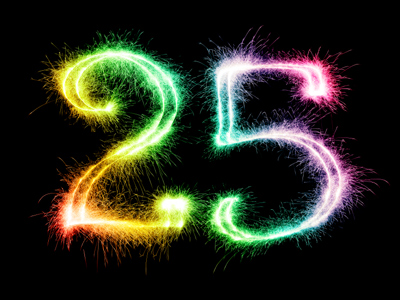Do you know the square root of 25? Find out in this quiz!

# Square Roots Part 2 - Imperfect Roots

This Math quiz is called 'Square Roots Part 2 - Imperfect Roots' and it has been written by teachers to help you if you are studying the subject at middle school. Playing educational quizzes is a fabulous way to learn if you are in the 6th, 7th or 8th grade - aged 11 to 14.

It costs only \$12.50 per month to play this quiz and over 3,500 others that help you with your school work. You can subscribe on the page at Join Us

Square roots are either “perfect” meaning the whole number has a squared number that, when multiplied by itself will equal the number, i.e., 9 is a perfect number because 3 x 3 = 9, or they are “imperfect” which means there is no number when multiplied by itself will equal the square root.

For example the number 15 has no number that when multiplied by itself will give you the number 15. Therefore, 15 is an “imperfect root”.

The math symbol that represents a square root is: “√”. So using the example above it would read:

√16 = 4

Because there is no actual solution to finding a square root, the use of calculators comes in handy. In fact, for this quiz you will find some imperfect square roots listed. To find the answer you will have to use a calculator.

1.
√11
3.13
3.31
3.5
3.53
11 is an imperfect square root so using a square root calculator we find that the number 3.3166248 x 3.3166248 = 11. We then shorten that to 3.31. Answer (b) shows the correct squared number
2.
√25
4
5
6
3
5 x 5 = 25
It can also be written as 52 = 25. Answer (b) shows the correct squared number
3.
√21
4.13
4.27
4.58
4.76
21 is an imperfect square root so using a square root calculator we find that the number 4.5825757 x 4.5825757 = 21. We then shorten that to 4.58. Answer (c) shows the correct squared number
4.
√14
7
3.2
3.5
3.74
14 is an imperfect square root so using a square root calculator we find that the number 3.7416574 x 3.7416574 = 14. We then shorten that to 3.74. Answer (d) shows the correct squared number
5.
√33
5.74
5.84
5.88
5.98
33 is an imperfect square root so using a square root calculator we find that the number 5.7445626 x 5.7445626 = 33. We then shorten that to 5.74. Answer (a) shows the correct squared number
6.
√27
4.59
4.67
4.83
5.19
27 is an imperfect square root so using a square root calculator we find that the number 5.1961524 x 5.1961524 = 27. We then shorten that to 5.19. Answer (d) shows the correct squared number
7.
√43
6.35
6.55
6.16
6.37
43 is an imperfect square root so using a square root calculator we find that the number 6.5574385 x 6.5574385 = 43. We then shorten that to 6.55. Answer (b) shows the correct squared number
8.
√121
11
13
12
17
11 x 11 = 121
It can also be written as 112 = 121. Answer (a) shows the correct squared number
9.
√64
8
16
32
14
8 x 8 = 64
It can also be written as 82 = 64. Answer (a) shows the correct squared number
10.
√105
9.87
10.12
10.24
10.41
105 is an imperfect square root so using a square root calculator we find that the number 10.2469508 x 10.2469508 = 105. We then shorten that to 10.24. Answer (c) shows the correct squared number
Author:  Christine G. Broome